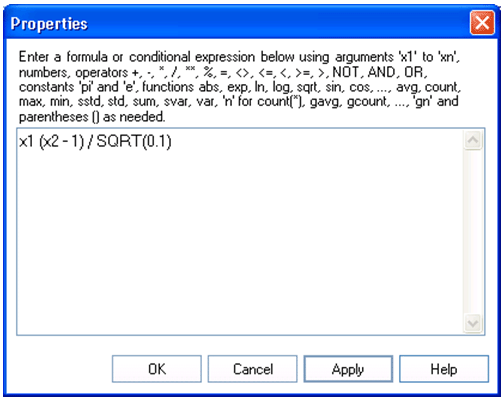# Formula - Teradata Warehouse Miner

Product
Release Number
5.4.6
Published
November 2018
Language
English (United States)
Last Update
2018-12-07
dita:mapPath
gxn1538171534877.ditamap
dita:ditavalPath
ft:empty
dita:id
B035-2301
Product Category
Software

An arithmetic formula or conditional expression with arguments may be entered using one of several argument formats.

 F(x1,..,xn) Any number of arguments: x1,…, xn where n is the number of arguments F(x) Zero or one argument: x F(x,y) Up to two arguments: x and y F(x,y,z) Up to three arguments: x, y and z

These SQL elements can be used anywhere a SQL expression can be created, such as in defining a Variable, Dimension, Expert Clause, ON Clause or Derive Transformation. In order to specify or edit a formula, simply highlight the formula element and click on Properties, or double-click the formula element. For example, if this is done with the element F(x1,...,xn), the following dialog appears.

Variable Creation > Input > Variables: SQL Elements pane - Other > Formula PropertiesIn the example above, the formula “x1 (x2 – 1) / SQRT(0.1)” is entered.

A Multiply operator ‘*’ is implied between the argument ‘x1’ and the left parenthesis ‘(‘, and that a leading 0 is used in specifying the constant value ‘0.1’, so that it is recognized as a number, as described in the rules below. Also, numbers should be entered in the current locale, so that ‘0.1’ should be entered as ‘0,1’ if comma is the locale’s decimal separator.
The following rules apply to formulas entered in the formula SQL elements.
1. Numbers must begin with a digit (‘0’ to ‘9’) and must be in integer, decimal or scientific formats according to current client locale settings.
2. Available operators include +, -, *, /, **, %, =, <>, <=, <, >=, >, NOT, AND, OR.
3. The numeric constant ‘pi’ and the exponential constant ‘e’ are available.
4. Available numeric functions include abs(), exp(), ln(), log(), sqrt(), sin(), cos(), tan(), asin(), acos(), atan(), sinh(), cosh(), tanh(), asinh(), acosh() and atanh().
5. Available aggregation functions include avg(), count(), max(), min(), sstd(), std(), sum(), svar(), var() and ‘n’ for number of rows, i.e., count(*).
‘sstd’ and ‘svar’ represent the sample standard deviation and sample variance functions respectively, whereas ‘std’ and ‘var’ represent the population version of these statistical functions. Also, count() represents the number of rows containing non-null values of the argument in parentheses, whereas ‘n’ or count (*) is simply the number of rows .
6. A Group style Windowed Aggregate variation of each aggregate function may be obtained by adding the letter ‘g’ to the front of each aggregate function name (gavg(), gcount(), gmax(), gmin(), gsstd(), gstd(), gsum(), gsvar(), gvar() and gn). These functions generate a Windowed Aggregate version of the underlying function with the Rows option specified in a Group style (i.e., ROWS BETWEEN UNBOUNDED PRECEDING AND UNBOUNDED FOLLOWING). This means that the aggregation is performed over all of the rows in the table regardless of any GROUP BY condition that may be in effect.

For example, the Z Score of an expression represented by the argument x1 is simply (x1 – GAVG(x1)) / GSTD(x1). Note that (x1 – AVG(x1)) / STD(x1) would receive the common error “Selected non-aggregate values must be part of the associated group” in a Variable Creation analysis. Also, because of the special handling of aggregates that a Derive transformation in a Variable Transformation analysis receives, both forms in this example would be successful in calculating the same result in a Derive transformation.

7. Parentheses may be used to control the order of evaluation.
8. Upper and lower case may be used interchangeably. For example, ‘x1’ is equivalent to ‘X1’, ‘pi’ is equivalent to ‘PI’, and so forth.
9. Whitespace characters are ignored.
10. Whenever a number, argument or right parenthesis is followed by an argument or left parenthesis, an implied multiply operator is automatically inserted in the generated SQL.
11. The operators in decreasing order of precedence are given below.
• Unary plus ‘+’ and minus ‘-‘
• Exponentiate ‘**’
• Multiply ‘*’, divide ‘/’ and modulo ‘%’
• Add ‘+’ and subtract ‘-‘
• Comparison ‘<=’, ‘<’, ‘>=’, ‘>’, ‘<>’, ‘=’
• ‘NOT’
• ‘AND’
• ‘OR’
12. If an SQL element other than one of the allowed elements is required in a formula, it must be entered as an argument and referred to in the formula by its argument name. For example, ‘x1’ might be used to represent the Cast of an expression.
13. Formulas may be nested by specifying a formula as the argument of another formula.